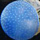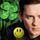Social QuestionAsked by LostInParadise (29296) December 4th, 2020

Imagine an addition table for the numbers 1 to 10. The rows and columns are both labeled from 1 to 10 and the entries in the table are row + column. What is the sum of all the entries in the table? You may find it helpful to use the fact that the sum of the numbers from 1 to 10 is 55. When I first thought of this, it seemed rather daunting, but the problem simplifies in a surprising way using some very elementary algebra.

Observing members: 0Composing members: 0After I solved the problem using algebra, I noticed the answer seemed very simple, and realized that there is a really easy way of solving it without algebra and without using the sum of the numbers from 1 to 10. Here is a hint on how to do it: Find a way of estimating the average value in the table. Show by simple reasoning that the estimate really is the average. Multiply that average by 100 to get the sum.

LostInParadise (29296)“Great Answer” (1) Flag as…I see no interest in the problem, so let me show why I think the solution is kind of interesting.

To estimate the average value, let’s look at the smallest value, at the top of the 1 column, 1+1, and the largest value 10+10 at the bottom of the 10 column, whose average is 22/2 = 11. We next go down the first column to 1+2 and up the last column to 10+9. Going down one column gives a number one larger, and going up the other column gives a number one smaller, so again we have an average of 11.

We can continue this way, moving down one column and up the other, until we simultaneously get to the bottom of the first column and the top of the second. Now what? We move to the right for the small number and to the left for the second. This again increases one number by 1 and decreases the other by 1, so the average is the same. Now we can again go up one column and down another, and keep going like this, moving horizontally when the top and bottom of the columns are reached.

We finally reach the bottom of the fifth column with value 5+10, and the top of the sixth column with 6+1, which still add to 22 and have an average of 11. Therefore the average of everything is 11, and the total must 11×100 = 1100.

LostInParadise (29296)“Great Answer” (1) Flag as…The above argument can be made more compact using symmetry.

Divide the table in half, either horizontally or vertically. The top left table value (1,1) will be in one half and the bottom right value (10,10) will be in the other half.

Any table value in the (1,1) half can be reached by starting at (1,1) and going a distance x to the right and y downward, where x or y may be 0. The value will be 1+x + 1+y.

There will be a corresponding value in the (10,10) half that is x to the left and y up from (10,10), whose value is 10-x + 10 -y.

In the first case the value of 2 is increased by x+y and in the second case the value of 20 is decreased by x+y, so when added we get 22. We have divided the table into pairs of points whose average is 11, and so the average of the whole table is 11, and again the sum of all the values is 11×100 = 1100.

LostInParadise (29296)“Great Answer” (2) Flag as…Yes, good puzzle.

If mathematics be the chemistry of love, play on.
Give me excess of it that, surfeiting,
The appetite may thicken, and so multiply.

doyendroll (1771)“Great Answer” (2) Flag as…Just for the sake of completeness, here is the original approach that I used. It is very straightforward but not very insightful.

I am going to let S= 1+2+3+...+10=55.
A quick way of computing it is to use the formula for arithmetic sequence.
1+2+3+...+n= n(n+1)/2 = 10×11/2=55.

Let’s add all the numbers in the first column. We are adding 1 to each of the numbers 1 to 10, so the first column sum is S + 10×1. Similarly the sum of the entries in the second column is S+10×2.

The sum of all 10 columns is (S + 10×1) + (S +10×2) + ... +(S+10×10) =
10S + 10(1+2+...+10) = 10S + 10S = 20S = 20×55 = 1100

LostInParadise (29296)“Great Answer” (1) Flag as…I tried to d it in my head but was unsuccessful. First column was simple. Then I moved to the next knowing the fixed increase , then the next but I had to cheat and use paper.
I love your “averaging” approach. Elegant.

LuckyGuy (40278)“Great Answer” (1) Flag as…